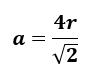# Problem: The density of an unknown metal is 12.3 g/cm3 and its atomic radius is 0.134 nm. It has a face-centered cubic lattice. Find the atomic mass of this metal.

###### FREE Expert Solution

Recall the equation used to calculate the edge length (a) of a Face-Centered Cubic (FCC) unit cell:Calculate edge length (a):

88% (48 ratings)###### Problem Details

The density of an unknown metal is 12.3 g/cm3 and its atomic radius is 0.134 nm. It has a face-centered cubic lattice. Find the atomic mass of this metal.